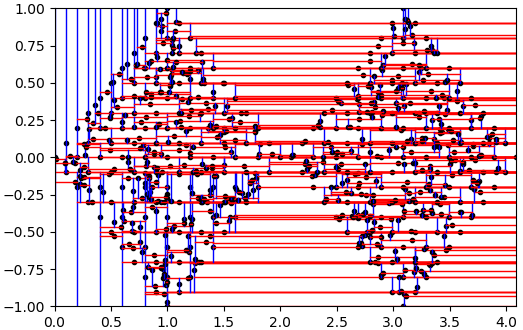pyclustering  0.10.1 pyclustring is a Python, C++ data mining library.
pyclustering.container.kdtree.kdtree Class Reference

Represents KD Tree that is a space-partitioning data structure for organizing points in a k-dimensional space. More...Inheritance diagram for pyclustering.container.kdtree.kdtree:Collaboration diagram for pyclustering.container.kdtree.kdtree:

## Public Member Functions

Create kd-tree from list of points and from according list of payloads. More...

Insert new point with payload to kd-tree. More...

def remove (self, point, **kwargs)
Remove specified point from kd-tree. More...Public Member Functions inherited from pyclustering.container.kdtree.kdtree_balanced
def __len__ (self)
Returns amount of nodes in the KD-tree. More...

def get_root (self)
Returns root of the tree. More...

Find node with specified coordinates and payload. More...

def find_node (self, point, cur_node=None)
Find node with coordinates that are defined by specified point. More...

def find_nearest_dist_node (self, point, distance, retdistance=False)
Find nearest neighbor in area with radius = distance. More...

def find_nearest_dist_nodes (self, point, distance)
Find neighbors that are located in area that is covered by specified distance. More...

## Detailed Description

Represents KD Tree that is a space-partitioning data structure for organizing points in a k-dimensional space.

In the term k-d tree, k denotes the dimensionality of the space being represented. Each data point is represented as a node in the k-d tree in the form of a record of type node. The tree supports dynamic construction when nodes can be dynamically added and removed. As a result KD-tree might not be balanced if methods insert and remove are used to built the tree. If the tree is built using constructor where all points are passed to the tree then balanced tree is built. Single point search and range-search procedures have complexity is O(n) in worse case in case of unbalanced tree. If there is no need to build dynamic KD-tree, then it is much better to use static KD-tree kdtree_balanced.

There is an example how to use KD-tree to search nodes (points from input data) that are nearest to some point:

# Import required modules
from pyclustering.samples.definitions import SIMPLE_SAMPLES;
from pyclustering.container.kdtree import kdtree;
# Read data from text file
# Create instance of KD-tree and initialize (fill) it by read data.
# In this case the tree is balanced.
tree_instance = kdtree(sample);
# Search for nearest point
search_distance = 0.3;
nearest_node = tree_instance.find_nearest_dist_node([1.12, 4.31], search_distance);
# Search for nearest point in radius 0.3
nearest_nodes = tree_instance.find_nearest_dist_nodes([1.12, 4.31], search_distance);
print("Nearest nodes:", nearest_nodes);

In case of building KD-tree using insert and remove method, the output KD-tree might be unbalanced - here is an example that demonstrates this:

from pyclustering.container.kdtree import kdtree, kdtree_visualizer
from pyclustering.samples.definitions import FCPS_SAMPLES
# Build tree using constructor - balanced will be built because tree will know about all points.
tree_instance = kdtree(sample)
kdtree_visualizer(tree_instance).visualize()
# Build tree using insert only - unbalanced tree will be built.
tree_instance = kdtree()
for point in sample:
tree_instance.insert(point)
kdtree_visualizer(tree_instance).visualize()

There are two figures where difference between balanced and unbalanced KD-trees is demonstrated.Fig. 1. Balanced KD-tree for sample 'TwoDiamonds'.Fig. 2. Unbalanced KD-tree for sample 'TwoDiamonds'.
kdtree_balanced

Definition at line 503 of file kdtree.py.

## ◆ __init__()

 def pyclustering.container.kdtree.kdtree.__init__ ( self, data_list = None, payload_list = None )

Create kd-tree from list of points and from according list of payloads.

If lists were not specified then empty kd-tree will be created.

Parameters
 [in] data_list (list): Insert points from the list to created KD tree. [in] payload_list (list): Insert payload from the list to created KD tree, length should be equal to length of data_list if it is specified.

Reimplemented from pyclustering.container.kdtree.kdtree_balanced.

Definition at line 569 of file kdtree.py.

## ◆ insert()

 def pyclustering.container.kdtree.kdtree.insert ( self, point, payload = None )

Insert new point with payload to kd-tree.

Parameters
 [in] point (list): Coordinates of the point of inserted node. [in] payload (any-type): Payload of inserted node. It can be ID of the node or some useful payload that belongs to the point.
Returns
(node) Inserted node to the kd-tree.

Definition at line 582 of file kdtree.py.

## ◆ remove()

 def pyclustering.container.kdtree.kdtree.remove ( self, point, ** kwargs )

Remove specified point from kd-tree.

It removes the first found node that satisfy to the input parameters. Make sure that pair (point, payload) is unique for each node, otherwise the first found is removed.

Parameters
 [in] point (list): Coordinates of the point of removed node. [in] **kwargs Arbitrary keyword arguments (available arguments: 'payload').

Keyword Args:

Returns
(node) Root if node has been successfully removed, otherwise None.

Definition at line 625 of file kdtree.py.

The documentation for this class was generated from the following file:
pyclustering.container.kdtree
Data Structure: KD-Tree.
Definition: kdtree.py:1
pyclustering.utils
Utils that are used by modules of pyclustering.
Definition: __init__.py:1